# Capacitor Mounting Surface

### Introduction

Choose the type of capacitor that has the longest life. · Estimate the average service life of the mounting surface capacitor under normal operating conditions:

• Tension : 50 Volts
• Température : 50° Celsus

### Evaluation method of the average life of the capacitor mounting surface.

Reliability is expensive. But the cost of poor quality and reliability is even more expensive. Reliability must be supported throughout the life cycle of the product. Predicting reliability is very important for electronic components such as capacitors, diodes and resistors.

To estimate this average lifetime of the surface-mount capacitor, a specific method of acceleration of lifetime is set up. Indeed the capacitors have a life of several years, making their tests very long. Generally the acceleration of the life tests are done by accelerating the failure of the product by the addition of sustained stress.

As part of this study, two non-controllable parameters are used: temperature and voltage. By stressing these two parameters, the life of the capacitor will be much less with reasonable failure times, and will allow an estimate of the service life under average conditions of use.

The value measured in these tests is the MTTF (Mean Time To Failure) which represents the average time of failure.

### Description of the model and the parameters of the study

The parameters of the study are:

• Y : Average operating time to failure (in h)
• 2 Production factors (controllable factors):
• A: The type of dielectric (composition of the dielectric). 2 levels A1 and A2.
• B: Operating temperature of the production process. 2 levels B1 and B2.
• 2 Environmental factors (non-controllable factors):
• C: Voltage (operating factors). 4 levels C1 = 200V, C2 = 250V, C3 = 300V and C4 = 350V.
• D: Ambient temperature (environmental factor): 2 levels D1 = 175 ° C and D2 = 190 ° C.

The mathematical model for the factors of production is the following one taking into account only the links of the second order for the factors A and B: We use the Taguchi method where we minimize the dispersion of product performance in response to noise factors while maximizing dispersion in response to signal factors. S / B ratios can be calculated using Taguchi’s robust plan options. There are three ratios:

• « Larger is better » to maximize the answer: S / N = -10 * log (Σ (1 / Y2) / n)
• « Smaller is better » to minimize the response: S / N = -10 * log (Σ (Y2) / n)
• « Nominal is best » based on mean and standard deviation

## Choice of factorial plans

### Production factors plan

L4 full factorial design plan for production factors:

 Dielectric Production temperature Durée de vie 1 1 1 2 2 1 2 2

The model on the factors of production is the following The model has 4 levels of freedom. With AB factor, the LCP is 4. For orthogonality, the number of tests of the plane must be multiple of k (4). And to respect the number of degrees of freedom, the number of tests must be at least 4.

### Experience Plan for Environmental Factors

Full L8 Factorial Experiment Plan for Environmental Factors: We begin by changing the Tension factor to 4 levels by two factors at two levels:

 Tension (V) 200 250 300 350
 Tension 1 Tension 2 1 1 1 2 2 1 2 1

The final experience plan is:

 Tension 1 Tension 2 Température Lifetime 1 1 175 1 1 190 1 2 175 1 2 190 2 1 175 2 1 190 2 2 175 2 2 190

Postulated Model: For orthogonality, the number of tests of the plane must be multiple of k (8). And to respect the number of degrees of freedom, the number of tests must be at least 8.

### Complete Factorial Plan with results

L4 + L8 Full Experience Plan Data

 Tension 1 1 2 2 3 3 4 4 Signal / Bruit Température  de fonction 1 2 1 2 1 2 1 2 Diélectrique Température de production Moyenne Ecart type Maximisation Valeur cible 1 1 430 950 560 210 310 230 250 230 396,25 2545 49,2 3,85 1 2 1080 1060 890 450 430 320 340 430 625 326,8 53,3 5,63 2 1 890 1060 680 310 310 310 250 230 505 325,5 50,5 3,8 2 2 1100 1080 1080 460 620 370 580 430 715 317,8 54,96 7,04

## Analysis of the reliability of the factors of production

### Model

Experience results for factors of production We take into account the average life span per group of factors, without considering the environmental factors directly.

 Dielectric Production temperature Durée de vie 1 1 396,25 1 2 625 2 1 505 2 2 715

### Analysis of the variance

To verify the conditions necessary for an analysis of variance, we must check the independence of the samples, the normality of the distribution and the homogeneity of the variances.

#### Normality of distribution

To test the normality of the distribution, we use a Shapiro-Wilk test. Statistic : 0.984044, Probability: 0,900591 . The probability of the test performed is greater than 5%, we can not reject the idea that the service life follows a normal distribution at the 95% level of confidence. The normality graph confirms the Gaussian distribution.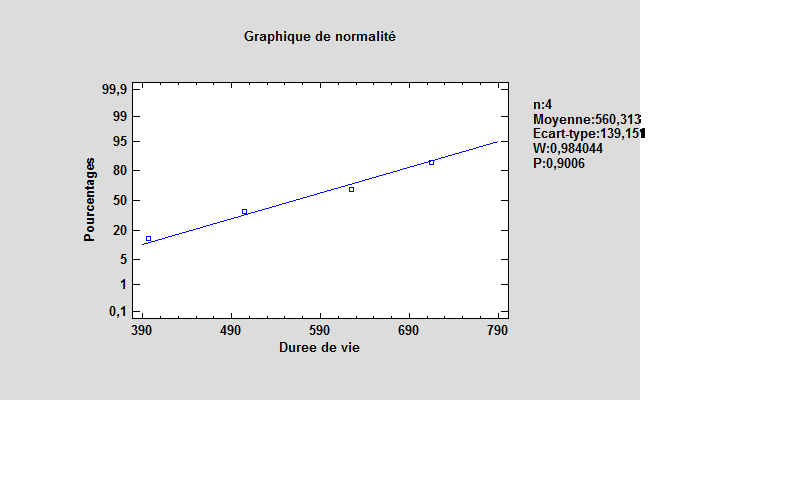#### Independence of samples

The plot of residuals by number of observations makes it possible to highlight the independence of the samples.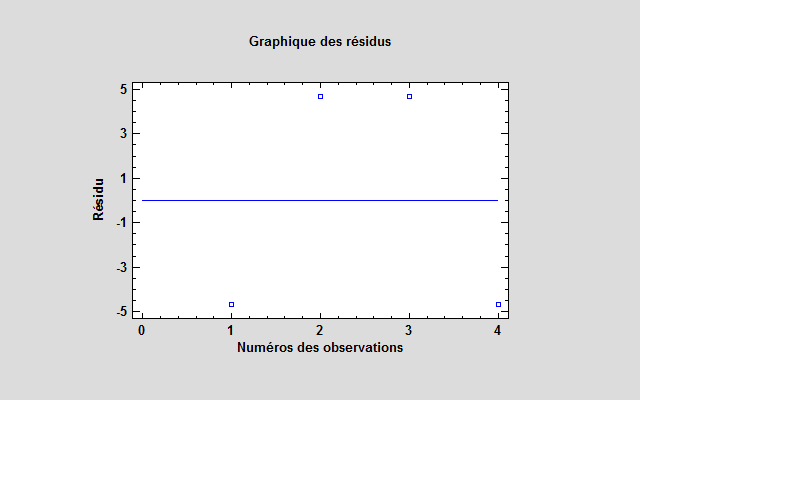#### Homogeneity of Variances

The plot of Residues by Production Temperature and Dielectric allows to conclude a homogeneous variance. The two graphs show residual variances of the same level.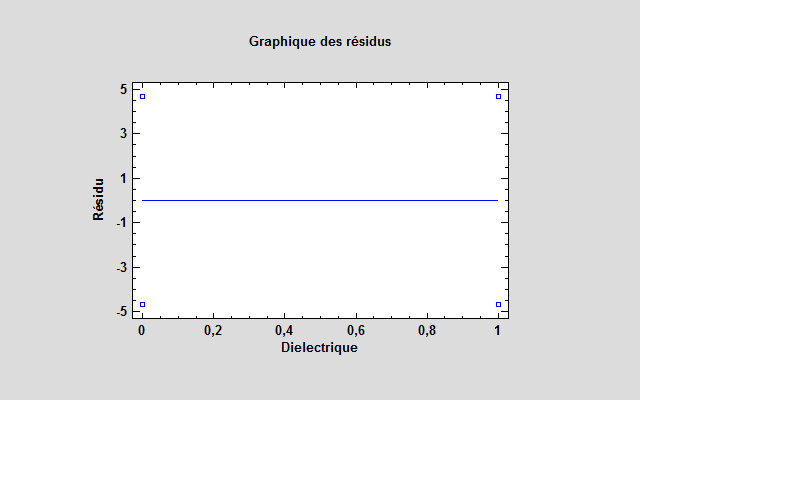#### Analysis of the ANOVA

Analysis with second order interactions.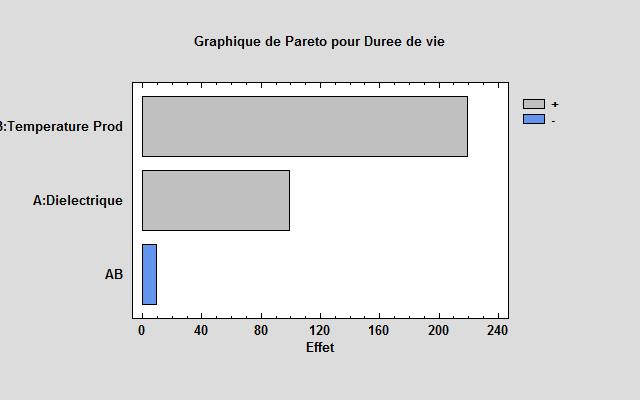The Pareto graph shows that the AB interaction is negligible. As part of the study, we will not consider this interaction. Analysis without AB interaction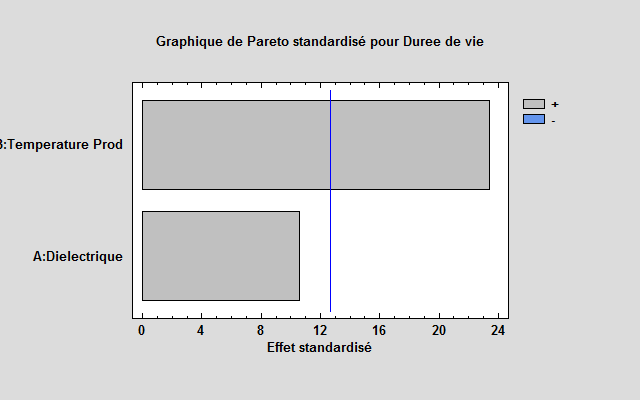By eliminating the AB interaction, we can evaluate the quality of each factor in the model. The results are as follows:

 Source Production Sum of squares DDL Mean quadratic Report F Proba. A:Dielectrique 9875,39 1 9875,39 112,36 0,0599 B:Température Production 48125,4 1 48125,4 547,56 0,0272 Total Error 87,8906 1 87,8906 Total (corr.) 58088,7 3

The effect of the production temperature has a probability of less than 5% which indicates that it is significantly different from zero and has a decisive effect at the 95% confidence level.The effects graph highlights the effect of each of the factors. It highlights and corroborates the analysis of the variance on the strong effect of the production temperature in the model. The effect of the dielectric is less important and can be neglected. The model adjusted with the dielectric factor is as follows: CONSTANT:560,313 , Diélectrique:49,6875 , Température Production:109,688. The equation of the adjusted model is: Durée de vie = 560,313 + 49,6875*Diélectrique + 109,688*Température de Production . The best combination that achieves the longest life is a dielectric factor of type 2 and a production temperature factor of level 2. This brings us to an estimated lifetime of 879.064 hours. The adjusted model without the dielectric factor is as follows: Durée de vie = 231,25 + 219,375*Température de Production. The best combination that achieves the longest life is a production temperature factor of 2. This brings us to an estimated life of 670 hours.

## Environmental factors analysis

### Signal / Noise Analysis

#### Results of experimentation

 Dielectric Production temperature S / B The biggest of the best S / B Target value 1 1 49,2 3,85 1 2 53,3 5,63 2 1 50,5 3,8 2 2 54,96 7,04

We choose « the greatest the best » and the target value: · For one hand to know the best combination acting on the service life. · And on the other hand, identify combinations that offer greater resistance to environmental factors.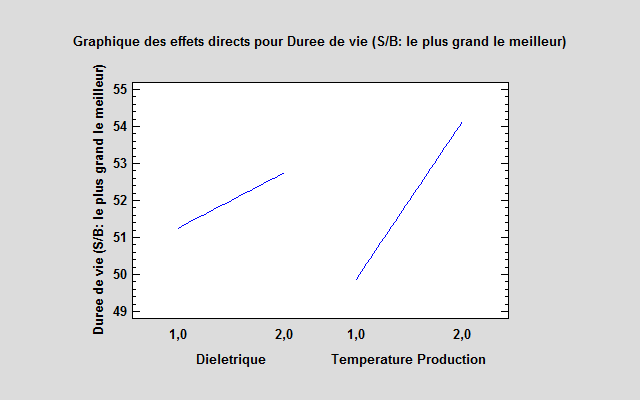The analysis of the values ​​and graphs of the effects makes it possible to choose the best combination respecting the instructions of robustness. Thus, the choice of the type 2 production temperature and the type 2 dielectric appears again as the best compromise.

### Study of noise factors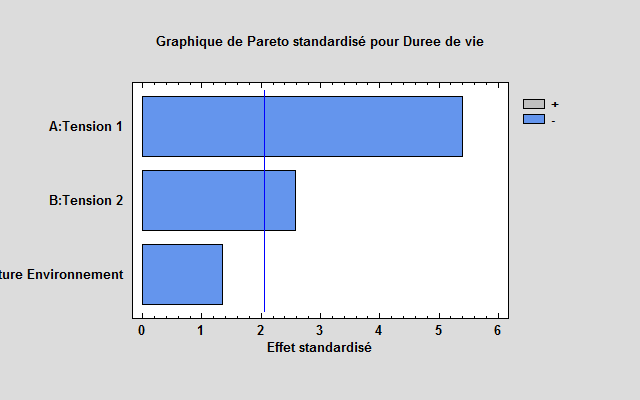{ » « } Voltage has a preponderant effect on the effect of noise in our model. The temperature factor of the environment is negligible in view of the Pareto graph. This is confirmed by the effects graph. The following variance analysis can also be checked:

 Source Sum of squares DDL Mean quadratic Report F Proba. A:Tension 1 1,38195E6 1 1,38195E6 29,07 0,0000 B:Tension 2 314028 1 314028 6,61 0,0158 C:Temperature Environnement 87153,1 1 87153,1 1,83 0,1866 Total Error 1,33116E6 28 47541,5 Total (corr.) 3,1143E6 31

## Estimated life expectancy under normal conditions

The data used is taken from the previous choice which is the Dielectric factor at Level 2 and the Production temperature factor at level 2.

 Tension Temperature Lifetime 200 175 1100 200 190 1080 250 175 1080 250 190 460 300 175 620 300 190 370 350 175 580 350 190 430

We use a survival data regression model with a normal log distribution. The result of the model estimation is as follows: Estimated Regression Model – Log-Normal The model is as follows: Duree Modele = exp(13,1167 – 0,00545509*Tension Env – 0,0281227*Temperature Env) . For a temperature of 50 ° and a voltage of 50 V, the estimate gives us: 92763 hours Which corresponds to 10 years of life. Model graph adjusted to 50V and 50 °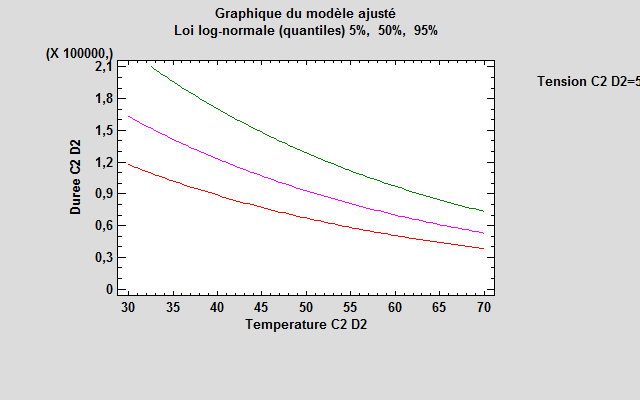## Conclusion

The analysis of the results allowed us to highlight the best compromise between the robustness and the longer life of surface response capacitors. Thus, it emerges from the foregoing elements, the surface capacitor having a type A2 dielectric and a type B2 production temperature. Based on this choice, we have determined, through a survival data regression model, that the surface response capacitor of this type has an estimated lifetime value of 92763 hours (10.6 years). Combined with the adjusted model graph, we found that the most robust of this type of surface response capacitor exceeded 130,000 hours (14.8 years).